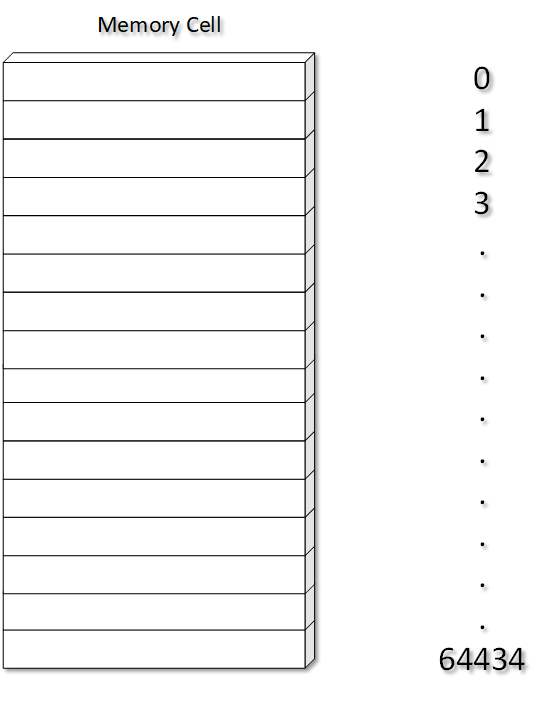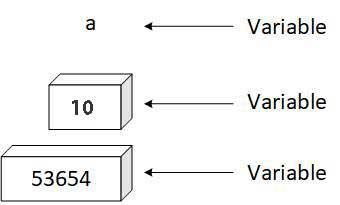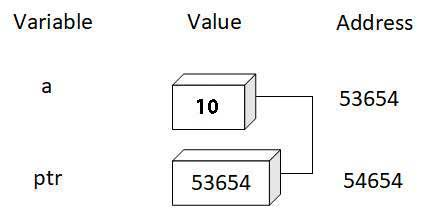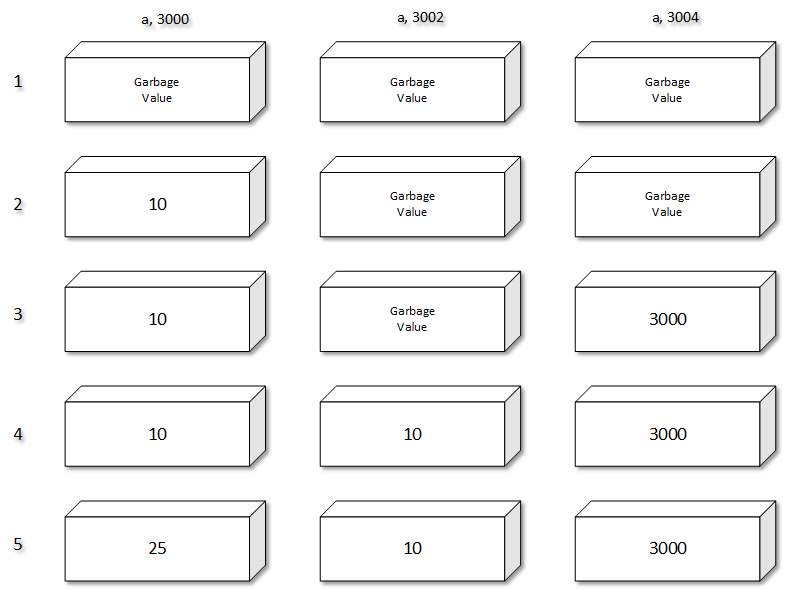# Pointer C Programing

Answer: The Computer’s memory is a sequential collection of 'storage cell’. Each cell, commonly known as a byte, has a number called address associate with it. The addresses are numbered consecutively, starting from zero. The last address depends on memory size. The memory organization is shown in following figure.Whenever we declare a variable, the system allocates an appropriate location to store the value of the variable.

For Example:

int a = 10;

will instruct the system to find a location for integer variable 'a’ and store the value 10 in that location.During execution we can access value 10 by using either the name ‘a’ or the address ‘52645’. As addresses are simple numbers, they can be stored in some variables. Such variables that hold memory addresses are called pointer variables.

A pointer variable is a variable that contains an address, which is a location of another variable in memory. Since a pointer is a variable, its value is also stored in another location.

For example, if a pointer variable ptr points to the variable 'a', then we can visualize it as in the memory.The following example illustrates. How we can declare and initialize a pointer variable And access the value of a variable using a pointer variable.

main ( )

{

int a,b, ptr;  /* declare 2 variables & 1 pointer vriable of type int */

a=10;       /* assign value 10 to a */

ptr= &a    /* Assign address of a to ptr */

b=*ptr    /* Assign value at ptr to b */

ptr=25   /* Assign 25 as vaule at ptr */

}

The above statement will be executed as follows:The pointer variable will take two bytes in memory.